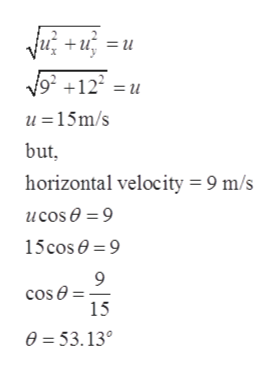# You can use any coordinate system you like to solve aprojectile motion problem. To demonstrate the truth of this statement, consider a ball thrown off the top of a building witha velocity vS at an angle u with respect to the horizontal. Letthe building be 50.0 m tall, the initial horizontal velocity be9.00 m/s, and the initial vertical velocity be 12.0 m/s. Chooseyour coordinates such that the positive y - axis is upward, thex - axis is to the right, and the origin is at the point where theball is released. (a) With these choices, find the ball’s maximumheight above the ground and the time it takes to reachthe maximum height. (b) Repeat your calculations choosingthe origin at the base of the building.

Question
32 views

You can use any coordinate system you like to solve a
projectile motion problem. To demonstrate the truth of this statement, consider a ball thrown off the top of a building with
a velocity vS at an angle u with respect to the horizontal. Let
the building be 50.0 m tall, the initial horizontal velocity be
9.00 m/s, and the initial vertical velocity be 12.0 m/s. Choose
your coordinates such that the positive y - axis is upward, the
x - axis is to the right, and the origin is at the point where the
ball is released. (a) With these choices, find the ball’s maximum
height above the ground and the time it takes to reach
the maximum height. (b) Repeat your calculations choosing
the origin at the base of the building.

check_circle

Step 1

Given:

Height of the building = 50 m

Initial horizontal velocity = 9 m/s

Initial vertical velocity = 12 m/s

Step 2

Resultant velocity can be calculated ashelp_outlineImage Transcriptioncloseu² +u, =u 9² +12² =u u =15m/s but, horizontal velocity = 9 m/s ucos 0 = 9 15cos 0 = 9 cos 0 = 15 0 = 53.13° fullscreen
Step 3

Part (a):

Let, maximum height covered by the ball = h

At maximum hei...

### Want to see the full answer?

See Solution

#### Want to see this answer and more?

Solutions are written by subject experts who are available 24/7. Questions are typically answered within 1 hour.*

See Solution
*Response times may vary by subject and question.
Tagged in

### Physics